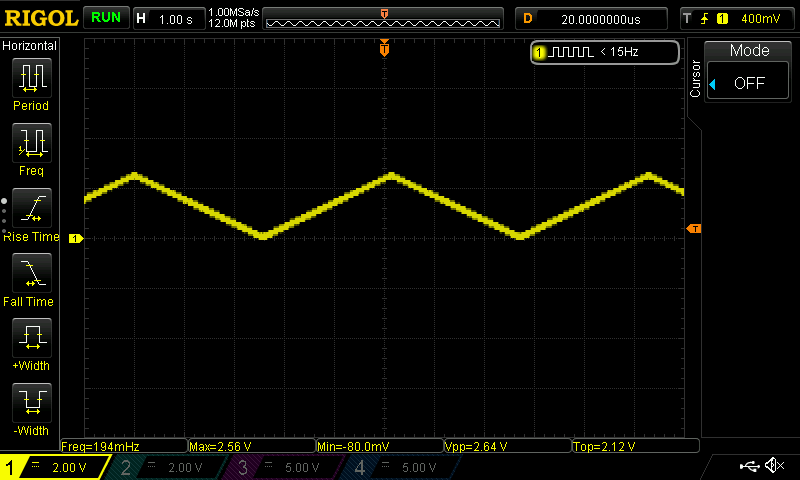# Using TinyCore¶

## GPIO¶

Follow the Arduino syntax, GPIO can be controlled as output or input.

Configured as output:

```pinMode(pin, OUTPUT);
digitalWrite(pin, HIGH);
```

You can check the pinout for reference:The Arduino Pin is marked as purple. To use a specific pin, for example:

```void setup()
{
pinMode(LED_BUILTIN , OUTPUT);
}

void loop() {
digitalWrite(LED_BUILTIN , HIGH);
delay(1000);
digitalWrite(LED_BUILTIN , LOW);
delay(1000);
}
```

## PWM¶

Same as Arduino, use analogWrite to generate PWM signals.

DAC PIN (or PIN 15) on TinyCore16/32 boards are true voltage output instead of PWM signals.

Simply use analogWrite on the DAC pin.:

```int val = 100; // (0 to 255)
analogWrite(LED_BUILTIN, val);
```

See DAC result below.PWM pins includes PIN 1, 2, 3, 12, 13, 14, use analogWrite on these pins to generate PWM signals:

```int val = 100;
analogWrite(1, val);
```

See PWM result below.Reference: https://www.arduino.cc/en/Tutorial/PWM

## ADC¶

There are 11 pins on TinyCore that support AnalogRead.

Available pins are marked in green color from A0 to A11. Some of the pins are in the extended pin area.

Use analogRead(A0) to read values from pins.

## DAC¶

TinyCore has 1 pin support real DAC output, which is pin 15.

The default DAC reference voltage is set as 2.5V.

You can change reference voltage in the board.txt file.

• Find -DDACVREF
• Use the lookup table below
• 0.55V -DDACVREF=0
• 1.1V -DDACVREF=1
• 1.5V -DDACVREF=4
• 2.5V -DDACVREF=2
• 4.3V -DDACVREF=3

Note: when power supply voltage is 3.3V, 4.3V reference is not working properly, you need to supply 5V to use 4.3V.

## Servo¶

Use Servo library like in Arduino. Servo should be available on all PWM pins (1,2,3,12,13,14).

## I2C¶

Fully Implement Wire library. SCL/SDA pins are marked on the board which is pin 3 and pin 2.

Simple Reader:

```#include <Wire.h>

void setup() {
Wire.begin();        // join i2c bus (address optional for master)
Serial.begin(9600);  // start serial for output
}

void loop() {
Wire.requestFrom(8, 6);    // request 6 bytes from slave device #8

while (Wire.available()) { // slave may send less than requested
char c = Wire.read(); // receive a byte as character
Serial.print(c);         // print the character
}

delay(500);
}
```

Simple Writer:

```#include <Wire.h>

void setup() {
Wire.begin(); // join i2c bus (address optional for master)
}

byte x = 0;

void loop() {
Wire.beginTransmission(8); // transmit to device #8
Wire.write("x is ");        // sends five bytes
Wire.write(x);              // sends one byte
Wire.endTransmission();    // stop transmitting

x++;
delay(500);
}
```

receiveEvent and requestEvent are also supported.

## UART¶

uart support up to 115200 baud rate.

To use uart, simply initialize it as:

```Serial.begin(9600);
```

Then you can send or receive data:

```Serial.println("test");
Serial.write(0x0d)
```

## SPI¶

Same as Arduino:

```#include <SPI.h>

byte address = 0;
byte value = 0x55;

void setup() {
SPI.begin();
}

void loop() {
SPI.transfer(address);
SPI.transfer(value);
}
```

Reference: https://www.arduino.cc/en/reference/SPI

## EEPROM¶

There are total 256 bytes eeprom on TinyCore 16/32 boards.

Usages are the same as Arduino:

```#include <EEPROM.h>

int val = EEPROM.read(addr);

EEPROM.write(addr, val);
```

Check example code for more details.

Now let’s use these to build awesome tiny projects!